# I.E. Irodov Solutions on Electromagnetic Waves Radiation

I.E. Irodov Solutions on chapter 4.4 Electromagnetic Waves (Radiation) contains solved examples and detailed answers to questions given in this unit. Students can refer to these solutions and develop better efficiency in tackling complex and difficult questions that students might come across while learning the different concepts. In order to make studying easier, the I.E. Irodov Solutions provided here offers the right knowledge that will further help them be proficient with the terminologies and concepts. In essences, students will be able to solve problems effectively and perform well in exams like IIT JEE amongst others.

### I.E. Irodov Solutions on Electromagnetic Waves Radiation

1: An electromagnetic wave of frequency v = 3.0 MHz passes from vacuum into a non-magnetic medium with permittivity a = 4.0. Find the increment of its wavelength.

Solution:

1. The velocity of light in a medium of relative permittivity c/√ε.

The change in wavelength of light (from its value in vacuum to its value in the medium) is

∆λ = (c/√ε)/v - c/v = c/v(1/√ε - 1) = -50 m

2: A plane electromagnetic wave falls at right angles to the surface of a plane parallel plate of thickness l. The plate is made of non-magnetic substance whose permittivity decreases exponentially from a value al at the front surface down to a value a, at the rear one. How long does it take a given wave phase to travel across this plate?

Solution:

1. From the data of the problem the relative permittivity of the medium varies as

ε(x) = ε1 e –(x/l)ln(ε1/ ε2)

Hence the local velocity of light

v(x) = c/ √ε(x) = [c/ √ε1] e –(x/2l)ln(ε1/ ε2)

Thus, the required time,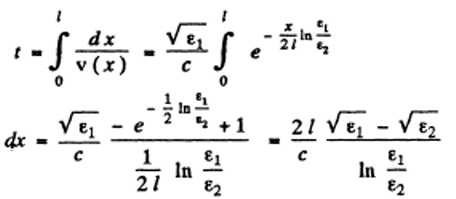3: A plane electromagnetic wave of frequency v = 10 MHz propagates in a poorly conducting medium with conductivity σ = 10 mS/m and permittivity ε = 9. Find the ratio of amplitudes of conduction and displacement current densities.

Solution:

1. Conduction current density= σE

Displacement current density:

\frac{\partial \vec{D}}{\partial t} = \varepsilon \varepsilon_0 \frac{\partial \vec{E}}{\partial t} = I \omega \varepsilon \varepsilon_0 \vec{E}

Ratio of magnitudes = σ/ωεε0 = 2

4: A plane electromagnetic wave E = Em cos (ωt-kr) propagates in vacuum. Assuming the vectors Em and k to be known, find the vector H as a function of time t at the point with radius vector r = 0.

Solution:

1.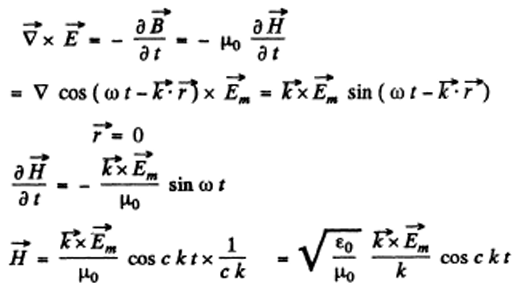[using ignoring the integration constant C and C= 1/√(εoμo)]

5: A plane electromagnetic wave E = Em cos (ωt-kr), where Em = Emey, k = kex, ex, eu are the unit vectors of the x, y axes, propagates in vacuum. Find the vector H at the point with radius vector r = xex at the moment (a) t = 0, (b) t = to. Consider the case when Em =1 60 V/m, k = 0.51 m-1, x = 7.7 m, and to = 33 ns.

Solution:

1. As in the previous problem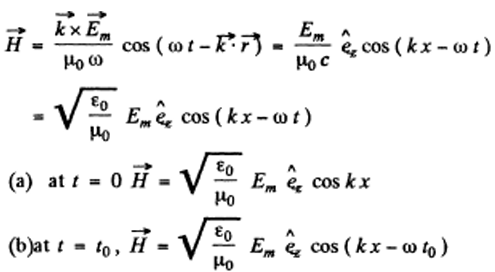6: A plane electromagnetic wave E = Em, cos (ωt-kx) propagating in vacuum induces the emf in, in a square frame with side l. The orientation of the frame is shown in Figure below. Find the amplitude value εind, Em =0.50m V/m, the frequency v = 5.0 MHz and l = 50 cm.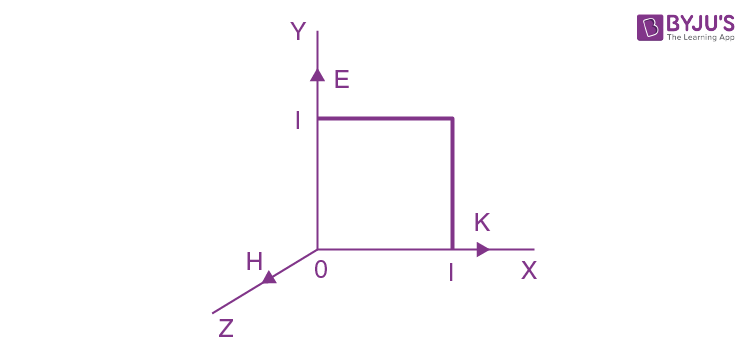Solution:

1.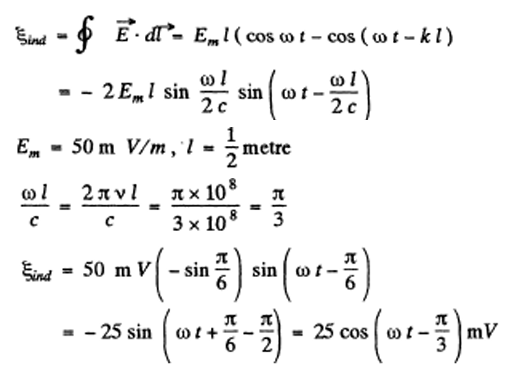7: Proceeding from Maxwell's equations show that in the case of a plane electromagnetic wave (fig below) propagating in vacuum the following relations hold:

\frac{\partial E}{\partial t}=-C^2 \frac{\partial B}{\partial x}, \frac{\partial B}{\partial t} = -\frac{\partial E}{\partial x}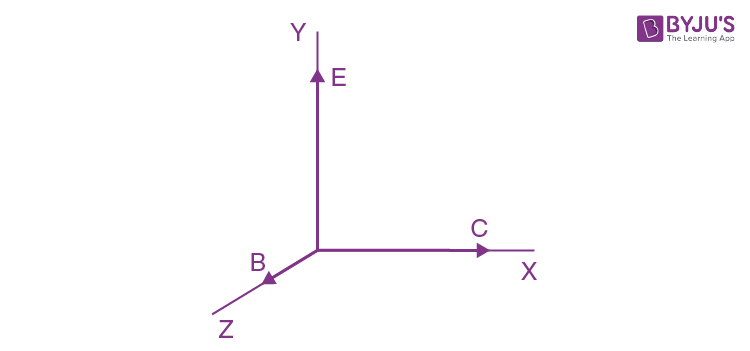Solution:

1.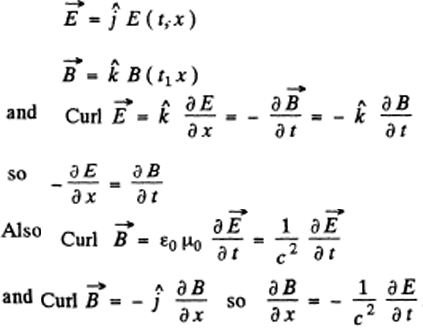8: Find the mean pointing vector (8) of a plane electromagnetic wave E = Emcos (ωt - kr) if the wave propagates in vacuum.

Solution:

1. \vec{E} = \vec{E_m}cos(\omega t - \vec{k}\vec{r})

\vec{H} = \sqrt{\frac{\epsilon_0}{\mu_0}} \frac{\vec{k}\times \vec{E_m}}{k}cos(\omega t - \vec{k}\vec{r})

So, \vec{S} = \vec{E} \times \vec{H} = \sqrt{\frac{\epsilon_0}{\mu_0}} \vec{E_m} \times (\vec{k} \times \vec{E_m} )(1/k) cos^2(\omega t - \vec{k}.\vec{r})

<\vec{S}> = (1/2) \sqrt{\frac{\epsilon_0}{\mu_0}} \vec{E_m^2} \frac{\vec{k}}{k}

9: A plane harmonic electromagnetic wave with plane polarization propagates in vacuum. The electric component of the wave has a strength amplitude Em = 50 mV/m, the frequency is v =100 MHz. Find:

(a) the efficient value of the displacement current density;

(b) the mean energy flow density averaged over an oscillation period.

Solution:

1. E = Em cos(2πvt – kx)

(a)jdis = partial derivative of D with t =2πε0vEm sin(ωt-kx)

Therefore,

(jdis)rms = < j2dis>1/2 = √2 πε0vEm = 0.20 mA/m2.

(b) <Sx> = (1/2) √(ε0o)Em2

So, <Sx> = 3.3 μ W/m2.

10: A ball of radius R = 50 cm is located in a non-magnetic medium with permittivity ε = 4.0. In that medium a plane electromagnetic wave propagates, the strength amplitude of whose electric component is equal to Em = 200 Vim. What amount of energy reaches the ball during a time interval t = 1.0 min?

Solution:

1. For the Pointing vector we can derive as in (196)

<s> = (1/2)√(εεoo)Em2

along the direction of propagation. Hence in time t (which is much longer than the time period T of the wave), the energy reaching the ball is

πR2 x (1/2)√(εεoo)Em2 x t = 5 kJ.

11: Figure below illustrates a segment of a double line carrying direct current whose direction is indicated by the arrows. Taking into account that the potential ϕ2 > ϕ1, and making use of the poynting vector, establish on which side (left or right) the source of the current is located.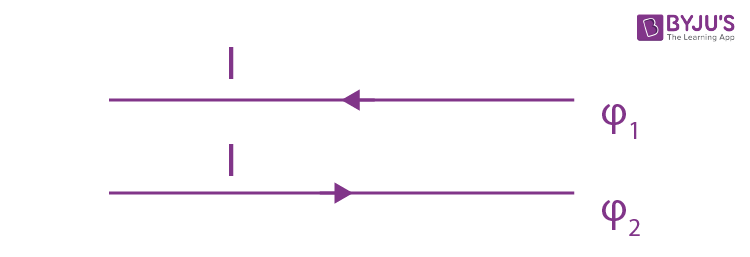Solution:

1. The electric field is as shown by dashed lines (->)

The magnetic field is as shown circles emerging out of the paper.

\vec{S}=\vec{E} \times \vec{H} is parallel to the wires and towards right.Hence source must be on the left.

12: Demonstrate that a closed system of charged non-relativistic particles with identical specific charges emits no dipole radiation.

Solution:

1.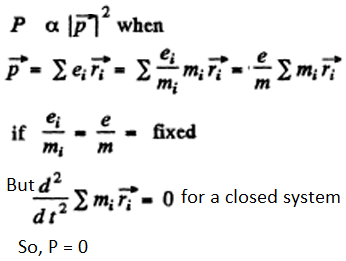13: Find the mean radiation power of an electron performing harmonic oscillations with amplitude a = 0.10 nm and frequency ω = 6.5.1014S-1.

Solution:

1. P = \frac{1}{4 \pi \epsilon_0}\frac{2 (\vec{P})^2}{3c^3}

|(\vec{P})^2|= (e \omega^2a)^2 cos^2 \omega t

Thus,<P> = \frac{1}{4 \pi \epsilon_0}\frac{2}{3c^3}( e \omega^2a)^2 \times 1/2

= 51. X 10-15 W.

14: Find the radiation power developed by a non-relativistic particle with charge e and mass m, moving along a circular orbit of radius R in the field of a stationary point charge q.

Solution:

1. Here

\vec{P} = \frac{e}{m} \times force =\frac{e^2q}{mR^2} \frac{1}{4 \pi \epsilon_0}

Thus, P = \frac{1}{(4 \pi \epsilon_0)^3} (\frac{e^2q}{mR^2})^2 \frac{2}{3c^3}

15: An electromagnetic wave emitted by an elementary dipole propagates in vacuum so that in the far field zone the mean value of the energy flow density is equal to So at the point removed from the dipole by a distance r along the perpendicular drawn to the dipole's axis. Find the mean radiation power of the dipole, pole's axis. Find the mean radiation power of the dipole.

Solution:

1. We know that

So(r) α 1/r2

At other angles S(r, θ) α sin2 θ

Thus, S(r, θ) = So(r) sin2 θ = So sin2 θ

= So x 4 πr2 x (2/3) = (8 π/3)S0r2.

16: The mean power radiated by an elementary dipole is equal to Po. Find the mean space density of energy of the electromagnetic field in vacuum in the far field zone at the point removed from the dipole by a distance r along the perpendicular drawn to the dipole's axis.

Solution:

1. Solution: From the previous problem.

P0 = (8πS0r2)/3

Or So = 3Po/8πr2

Therefore, <w> = S0/c = 3Po/8πcr2

17: An electric dipole whose modulus is constant and whose moment is equal to p rotates with constant angular velocity w about the axis drawn at right angles to the axis of the dipole and passing through its midpoint. Find the power radiated by such a dipole.

Solution:

1. The rotating dispole has moments

px = p cos ωt, py = p sin ωt

P = \frac{1}{4 \pi \epsilon_0}\frac{2}{3c^3} \omega^4 p^2 =\frac{p^2\omega^4}{6\pi \epsilon_0c^3}

18: A free electron is located in the field of a plane electromagnetic wave. Neglecting the magnetic component of the wave disturbing its motion, find the ratio of the mean energy radiated by the oscillating electron per unit time to the mean value of the energy flow density of the incident wave.

Solution:

1. If the electric field of the wave

\vec{E} = \vec{E_0}cos \omega t

Then this induces a dipole moment whose second derivative is

\vec{\ddot{p}} = \frac{e^2 \vec{E_0}}{m}cos \omega t

<p> = (1/4πε0)(2/3c3)(e2E0/m)2 (1/2)

On the other hand, the mean Thus Pointing flux of the incident radiation is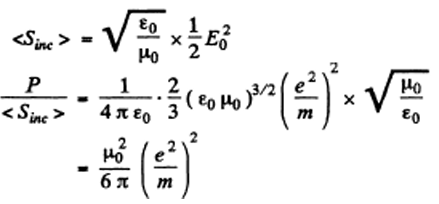19: A plane electromagnetic wave with frequency ω falls upon an elastically bonded electron whose natural frequency equals ωo. Neglecting the damping of oscillations, find the ratio of the mean energy dissipated by the electron per unit time to the mean value of the energy flow density of the incident wave.

Solution:

1. For the elastically bound electron

m \vec{\ddot{r}}+m \omega_0^2 \vec{r} =e \vec{E_o} cos \omega t

This equation has the particular integral (i.e. neglecting the part which does not have the frequency of the impressed force)

\vec{r}=\frac{e \vec{E_0}}{m} \frac{cos \omega t}{\omega_0^2 - \omega^2}

and, \vec{\ddot{p}} = - \frac{e^2 \vec{E_0} \omega^2}{(\omega^2_0-\omega^2)m} cos \omega t

Hence, P = mean radiated power

=\frac{1}{4 \pi \epsilon_0} \frac{2}{3C^3}(\frac{e^2\omega^2}{(\omega^2_0-\omega^2)m })^2

The mean incident pointing flux is

<Sinc> = √(εoo) (1/2) Eo2

Therefore, P/<Sinc> = (μo /6 π) (e2/m)24/( ω20 - ω2)2)

20: Assuming a particle to have the form of a ball and to absorb all incident light, find the radius of a particle for which its gravitational attraction to the Sun is counterbalanced by the force that light exerts on it. The power of light radiated by the Sun equals P = 4 x1026 W, and the density of the particle is ρ = 1.0 g/cm3.

Solution:

1. Let r = radius of the ball

R = distance between the ball and the Sun (r <<R).

M = mass of the Sun

y = gravitational constant Then

\frac{\gamma M}{R^2} \frac{4 \pi}{3} r^3 \rho = \frac{P}{4 \pi R^2}\pi r^2 (1/c)

(The factor 1/c converts the energy received on the right into momentum received. Then the right hand side is the momentum received per unit time and must equal to the negative of the impressed force for equilibrium).

Therefore, r = \frac{3P}{16 \pi \gamma M c \rho} = 0.606 \mu m